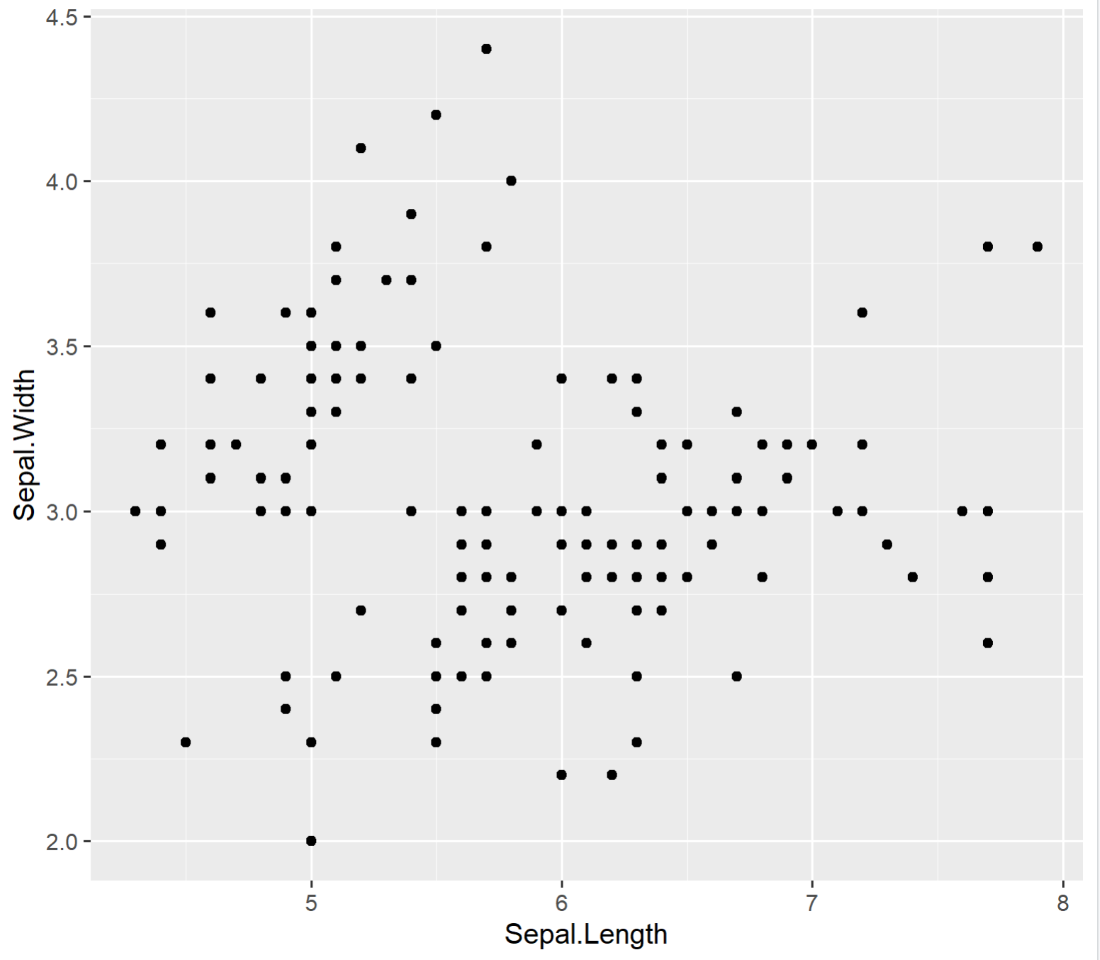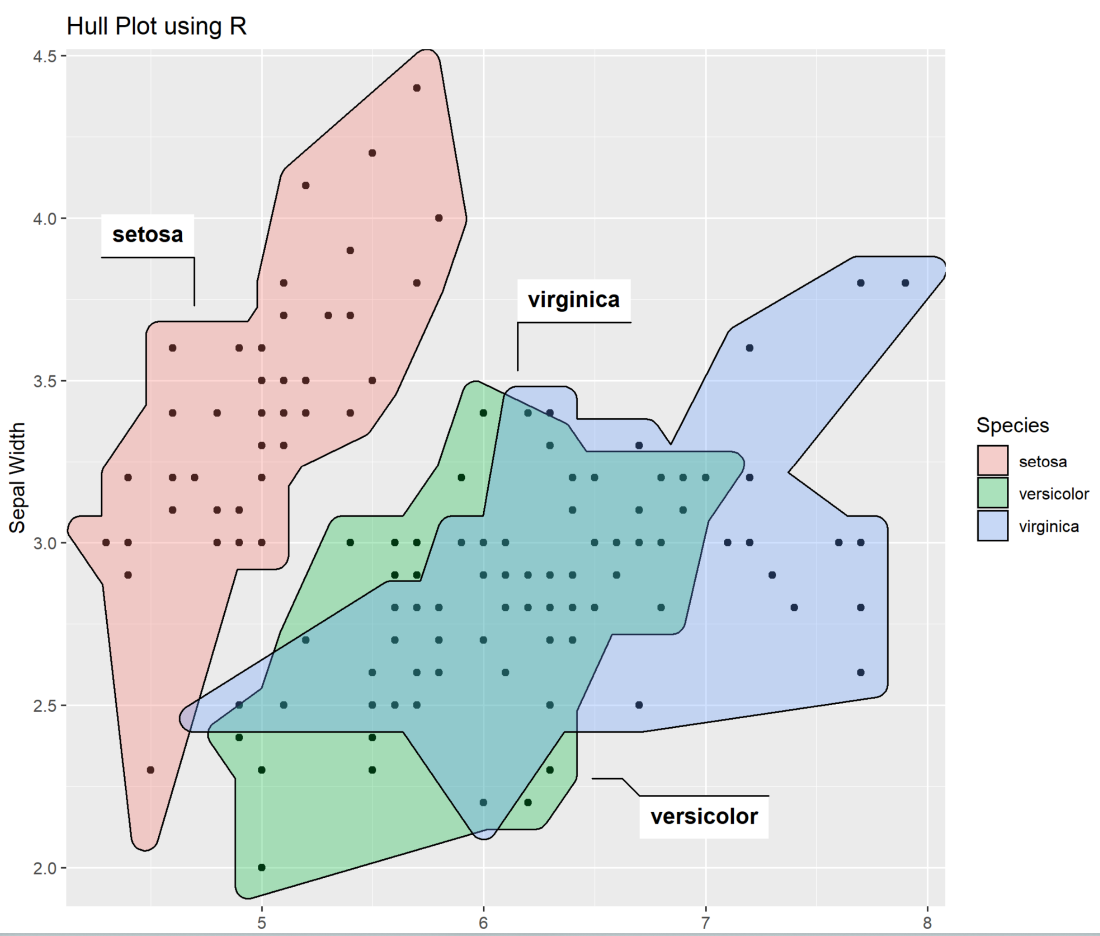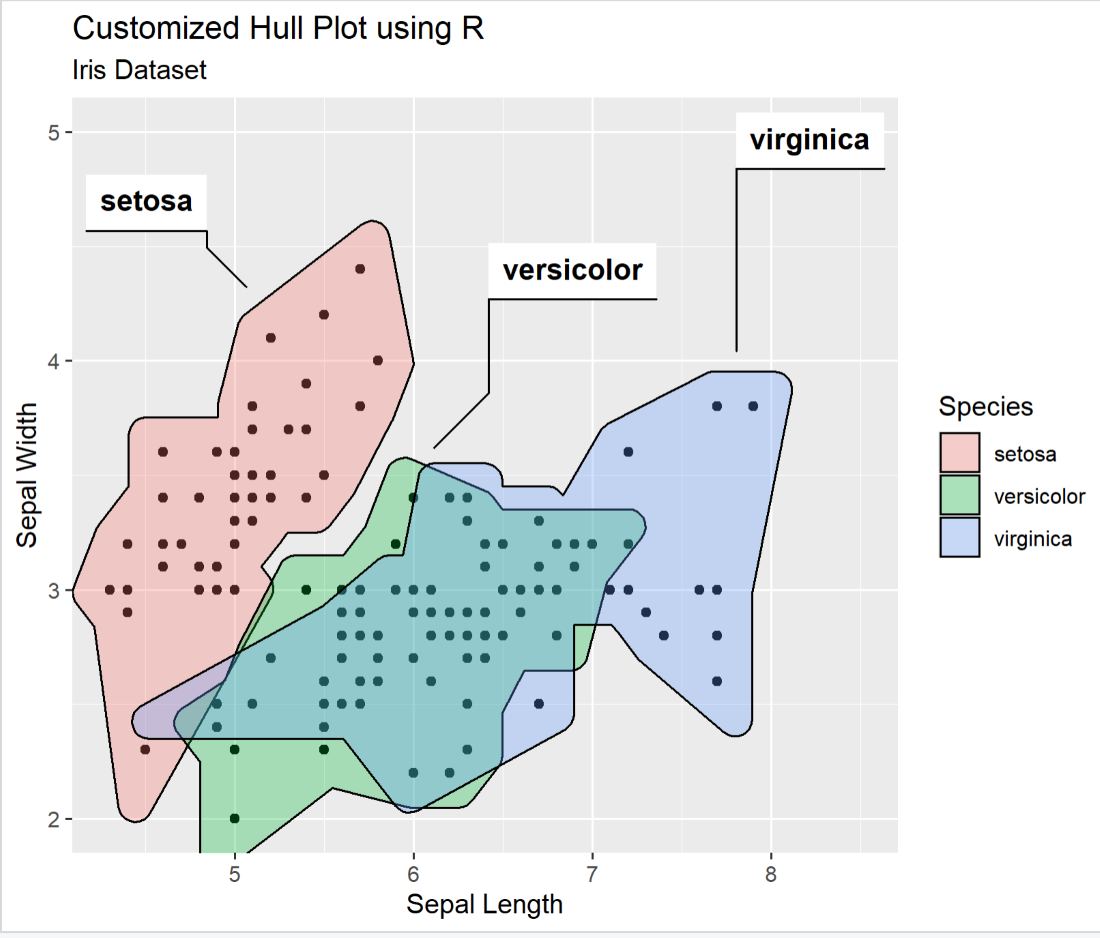GeeksforGeeks App
Open AppBrowser
Continue

# Visualizing clusters using Hull Plots in ggplot2 using ggforce

The HULL Plots are also known as Grouped Scatter Plots because these plots are used to segregate the scatter plots based on clusters. The Hull plots are much more useful when one wants to visualize the clusters present among the data. The Hull plots in R can be plotted using the geom_mark_hull() function which is present in ggforce package.

### Syntax of geom_mark_hull() method

Where,

• mapping – set of aesthetic mappings created by aes() function
• data – The data frame to be displayed
• concavity – used to set the concavity of thehull
• position – used to adjust the position.

### Steps to Visualize Clusters using Hull Plot

Step 1: First, we need to install the required packages (ggplot2, ggforce) and load them.

## R

 `# Install Required Packages``install.packages``(``"ggplot2"``)``install.packages``(``"ggforce"``)` `# Load the installed Packages``library``(ggplot2)``library``(ggforce)`

Step 2:  Next we need to plot the basic scatter plot using ggplot() function in the ggplot2 package.

## R

 `# Load the default dataset (iris)``data``(iris)` `# Plotting the scatter plot using ggplot2 package``fig1<-ggplot2::``ggplot``(aes``                      ``(Sepal.Length,Sepal.Width),``                      ``data=iris)+``geom_point``()``print``(fig1)`

Output:Step 3: Later we will add hulls(clusters) to that scatter plot using geom_mark_hull() function in ggforce package.

## R

 `# Load the default dataset (iris)``data``(iris)` `# Plotting the scatter plot using ggplot2 package``fig1<-ggplot2::``ggplot``(``aes``(Sepal.Length,Sepal.Width),data=iris)+``geom_point``()` `# Plotting the Hull Plot using ggforce package``fig2 <- fig1 + ggforce::geom_mark_hull``    ``(``aes``(fill=Species,label=Species),``             ``concavity=2)` `print``(fig2)`

Output:Step 4:  We can also customize the Hull Plot in R using labs() function of ggforce package to add labels to the plot and using expand_limits() we can expand the limits of the x and y axes values that need to be considered to plot the graph.

## R

 `# Load the default dataset (iris)``data``(iris)` `# Plotting the scatter plot using ggplot2 package``fig1<-ggplot2::``ggplot``(aes``   ``(Sepal.Length,Sepal.Width),data=iris)+``geom_point``()` `# Plotting the Hull Plot using ggforce package``fig2 <- fig1 + ggforce::``geom_mark_hull``(aes``          ``(fill=Species,label=Species),concavity=2)` `# Customized Hull Plot``fig3 <- fig2 + ggforce::geom_mark_hull``    ``(``aes``(fill=Species,label=Species),concavity=2)+``       ``expand_limits``(x=8.5,y=5.0)+``       ``labs``(title=``"Customized Hull Plot using R"``,``            ``subtitle = ``"Iris Dataset"``,``            ``x=``"Sepal Length"``,y=``"Sepal Width"``)` `print``(fig3)`

Output:My Personal Notes arrow_drop_up